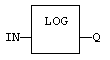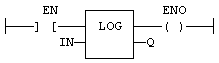# LOG

Function - Calculates the logarithm (base 10) of the input.

## Inputs

IN : REAL  Real value

## Outputs

Q : REAL   Result: logarithm (base 10) of IN

## Remarks

In LD language, the operation is executed only if the input rung (EN) is TRUE. The output rung (ENO) keeps the same value as the input rung. In IL, the input must be loaded in the current result before calling the function.

Q := LOG (IN);

## FBD Language## LD Language

(* The function is executed only if EN is TRUE *)
(* ENO keeps the same value as EN *)## IL Language:

Op1: LD  IN
LOG
ST  Q    (* Q is: LOG (IN) *)